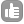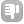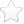0Hi! is there a possibility to perform an integer division or a modulo calculation in topspin 2.1? Thank you! asked Sep 06 '11 at 06:03guma 10Yes this is possible. It is mostly used in conditional statements. For example: if "l1 %2 == 1" would be true if l1 is odd and false if l1 is even. Integer division is also possible you can divide a loopcounter (integer) by another integer and the result in Topspin2.1 will be the truncated number. I.e l1 = 5 then l1/2 = 2. Contact me directly if you have more questions. Clemens answered Sep 06 '11 at 11:47